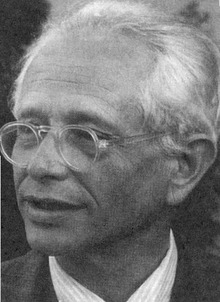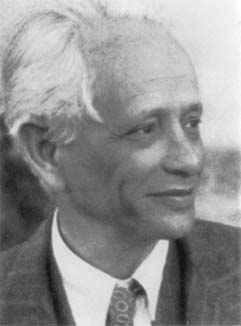# on Geometry, Topology, Dynamics, and Groups

### Academic year 2009 - 2010

 Date Speaker Title — click for abstract (if available) September 2, 2009 Jing Tao University of Utah Linearly bounded conjugator property for mapping class groups Given two conjugate mapping classes $$f$$ and $$g$$, we produce a conjugating element $$w$$ such that $$|w| \le K (|f| + |g|)$$, where $$|\cdot|$$ denotes the word metric with respect to a fixed generating set, and $$K$$ is a constant depending only on the generating set. As a consequence, the conjugacy problem for mapping class groups is exponentially bounded. September 9, 2009 Mladen Bestvina University of Utah Asymptotic dimension of Mapping class groups The first talk will review the concept of asymptotic dimension and some background material. In the second talk I will construct (many) actions of mapping class groups on quasi-trees, and show how this implies that mapping class groups have finite asymptotic dimension. This is joint work with Ken Bromberg and Koji Fujiwara. September 23, 2009 Martin Deraux University of Grenoble I (Institut Fourier) Pinching questions for manifolds of negative curvature September 30, 2009 Mladen Bestvina University of Utah Asymptotic dimension of Mapping class groups, cont. The first talk will review the concept of asymptotic dimension and some background material. In the second talk I will construct (many) actions of mapping class groups on quasi-trees, and show how this implies that mapping class groups have finite asymptotic dimension. This is joint work with Ken Bromberg and Koji Fujiwara. October 7, 2009 Christopher Cashen University of Utah Mapping Tori of Free Group Automorphisms and Line Patterns in Free Groups I will talk about line patterns in free groups and how they provide quasi-isometry invariants for mapping tori of linearly growing free group automorphisms. This is joint with Natasha Macura. October 28, 2009 William Malone University of Utah Isometries of Products of Uniquely Geodesic Metric Spaces with the Sup Norm are Reducible Let f be an isometry between spaces which are products of uniquely geodesic metric spaces with the sup norm. There are two obvious types of isometries from such a space to itself namely a permutation of the factor spaces and a product of isometries of the factor spaces. In this talk we will show that not only is the number of factor spaces an isometry invariant, but also that any isometry is a composition of the two isometries types mentioned above. November 11, 2009 Yael Algom-Kfir University of Utah Asymmetry of Outer Space The Lipschitz metric on Outer Space is not symmetric. In fact d(x,y)/d(y,x) can be arbitrarily large. In joint work with Mladen Bestvina, we define a piecewise differentiable function \psi on Outer Space (which is invariant under the action of Out(Fn) and show that d(x,y) can be bounded in terms of d(y,x) and \psi(x) - \psi(y). I will discuss the proof of this theorem and some applications. November 18, 2009 Kevin Wortman University of Utah Non-nonpositive curvature of some non-cocompact arithmetic groups I'll explain why arithmetic groups of relative Q-type A_n, B_n, C_n, D_n, E_6, and E_7 satisfy an exponential isoperimetric inequality in some dimension. December 2, 2009 Mladen Bestvina University of Utah A new proof of the train track theorem January 13, 2010 Kasra Rafi University of Oklahoma Lengths of curves along a Teichmüller geodesic We show that the extremal length and the hyperbolic length of any simple closed curve are quasi-convex functions of time along any Teichmüller geodesic. As a corollary, we conclude that, in Teichmüller space equipped with the Teichmüller metric, balls are quasi-convex. (Joint work with Anna Lenzhen.) January 27, 2010 Juan Souto University of Michigan (Non)-actions of the mapping class group on the unit tangent bundle. By the work of Morita and Markovic, it is known that the mapping class group of a surface $S$ does not act naturally on $S$. However, such an action, by Hoelder homeomorphisms, exists on the unit tangent bundle $T^1S$ of the surface. In this talk I will explain why this last action is conjugated to a Lipschitz action but not even homotopic to a smooth one. January 29, 2010 Alexandra Pettet University of Michigan Periodic maximal flats are not peripheral I will prove that every finite volume locally symmetric space contains a compact set K with the property that no periodic maximal flat can be homotoped to be disjoint from K. This is joint work with Juan Souto. February 3, 2010 Jeno Szigeti Miskolc, Hungary Centralizers in Endomorphism Rings February 10, 2010 Jing Tao University of Utah Teichmüller diameter of the thick part of moduli space Let S be a closed surface of genus $$g \ge 2$$. The thick part of the moduli space of S is the set of hyperbolic metrics on S such that the length of the shortest loop is bounded below by a fixed constant. We study the asymptotic behavior of the diameter of this set equipped with the Teichmüller metric and prove that it grows like $$\log(g)$$. This is joint with Kasra Rafi. March 3, 2010 William Malone University of Utah Quasi-isometric Classification of Geometric Amalgamations of Free Groups In order to understand the large scale geometry of limit groups a good starting place is with geometric amalgamations of free groups (a class of graphs of groups) since they are virtually limit groups. In this talk a complete quasi-isometric invariant for geometric amalgamations of free groups will be given, along with an elementary example showing that commensurability and quasi-isometry are not the same equivalence relation for virtual limit groups. March 5, 2010 John Parker Durham Constructing non-arithmetic lattices The first examples of non-arithmetic complex hyperbolic lattices were given by Mostow in 1980. These examples are generalised triangle groups generated by complex reflections of orders 3, 4 or 5. I will discuss how to parametrise such triangle groups and how to identify which of them may possibly be lattices. Most of these candidates are non-arithmetic. I will then survey an ongoing project with Deraux and Paupert whose goal is to use this idea to construct (families of) new non-arithmetic complex hyperbolic lattices. March 10, 2010 David Futer Temple University Bourdon's building and hyperbolic surfaces Bourdon's building is a negatively curved 2-complex built out of hyperbolic right-angled polygons. Its automorphism group is large (uncountable) and remarkably rich. We study, and mostly answer, the question of when there is a discrete subgroup of the automorphism group such that the quotient is a closed surface of genus g. This involves some fun elementary combinatorics, but quickly leads to open questions in group theory and number theory. This is joint work with Anne Thomas. March 17, 2010 Anne Thomas University of Oxford Lattices in complete Kac-Moody groups A complete Kac-Moody group over a finite field is a totally disconnected, locally compact group, which may be thought of as an "infinite-dimensional Lie group". We study cocompact lattices in such groups of rank 2, where the associated building is a tree, using the group action on the tree and finite group theory. This is joint work with Inna (Korchagina) Capdeboscq. April 14, 2010 Tim Riley Cornell Hydra Groups I will describe some wild geometry that arises in an apparently benign group theoretic setting: I will exhibit a family of groups enjoying a number of restrictive geometric and algebraic conditions (they are CAT(0), bi-automatic, 1-relator, and free-by-cyclic), and yet these groups have free subgroups of huge (Ackermannian) distortion. The origin of this behaviour lies in a simple computational game --- a realisation of Hercules' battle with the hydra, played out in manipulations of strings of letters. This is work with Will Dison. May 5, 2010 Erika Meucci University of Utah Relative Outer Automorphisms of Free Groups In this talk I will describe a (contractible) relative outer space on which the group of relative outer automorphisms of a free group acts properly and discontinuously.

Current seminar          Archive of past talks
Max Dehn Seminar is organized by Mladen Bestvina, Ken Bromberg, Patrick Reynolds,
Jing Tao, Domingo Toledo, and Kevin Wortman.

This web page is maintained by Patrick Reynolds and Jing Tao.